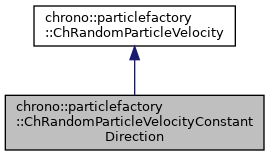chrono::particlefactory::ChRandomParticleVelocityConstantDirection Class Reference

Description

Generator of random particle velocities with constant direction.

Modulus is constant by default, too, but it can be set as randomized via a statistical distribution.

#include <ChRandomParticleVelocity.h>

Inheritance diagram for chrono::particlefactory::ChRandomParticleVelocityConstantDirection:[legend]
Collaboration diagram for chrono::particlefactory::ChRandomParticleVelocityConstantDirection:[legend]

Public Member Functions

virtual ChVector RandomVelocity () override
Function that creates a random velocity each time it is called. More...

void SetDirection (ChVector<> mdir)
Set the direction for all the randomized velocities.

void SetModulusDistribution (std::shared_ptr< ChDistribution > mdistr)
Set the statistical distribution for the modulus of velocities.

void SetModulusDistribution (double mval)
Set the modulus of velocities as a constant value.

Member Function Documentation

 virtual ChVector chrono::particlefactory::ChRandomParticleVelocityConstantDirection::RandomVelocity ( )
overridevirtual

Function that creates a random velocity each time it is called.

Reimplemented from chrono::particlefactory::ChRandomParticleVelocity.